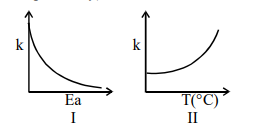# Consider the given plots for a reaction obeying

Question:

Consider the given plots for a reaction obeying Arrhenius equation $\left(0^{\circ} \mathrm{C}<\mathrm{T}<300^{\circ} \mathrm{C}\right)$ : $(\mathrm{k}$ and $\mathrm{E}_{\mathrm{a}}$ are rate constant and activation energy, respectively)Choose the correct option :

1. Both I and II are wrong

2. I is wrong but II is right

3. Both I and II are correct

4. I is right but II is wrong

Correct Option: , 4

Solution:

On increasing $E_{a}, K$ decreases